1.2. Photoionization Equilibrium

At every point in a optically thin, pure hydrogen medium of neutral density nHI, the photoionization rate per unit volume is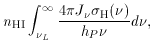(4)

where Jis the mean intensity of the ionizing radiation (in energy units per unit area, time, solid angle, and frequency interval), and hP is the Planck constant. The photoionization cross-section for hydrogen in the ground state by photons with energy hP(above the threshold hPL = 13.6 eV) can be usefully approximated by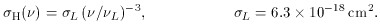(5)

At equilibrium, this is balanced by the rate of radiative recombinations p + e -> H +per unit volume,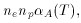(6)

where ne and np are the number densities of electrons and protons, andA =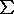<n ve > is the radiative recombination coefficient, i.e. the product of the electron capture cross-sectionn and the electron velocity ve, averaged over a thermal distribution and summed over all atomic levels n. At the commonly encountered gas temperature of 104 K,A = 4.2 x 10-13 cm3 s-1.

Consider, as an illustrative example, a point in an intergalactic H II region at (say) z = 6, with densityH = (1.6 x 10-7 cm-3) (1 + z)3 = 5.5 x 10-5 cm-3. The H II region surrounds a putative quasar with specific luminosity L= 1030 (L /)2 ergs s-1 Hz-1, and the point in question is at a distance of r = 3 Mpc from the quasar. To a first approximation, the mean intensity is simply the radiation emitted by the quasar reduced by geometrical dilution,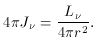(7)

We then have for the photoionization timescale: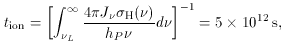(8)

and for the recombination timescale: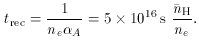(9)

As in photoionization equilibrium nHI / tion = np / trec, these values imply nHI / np10-4, that is, hydrogen is very nearly completely ionized.

A source radiating ultraviolet photons at a finite rate cannot ionize an infinite region of space, and therefore there must be an outer edge to the ionized volume (this is true unless, of course, there is a population of UV emitters and all individual H II regions have already overlapped). One fundamental characteristic of the problem is the very small value of the mean free path for an ionizing photon if the hydrogen is neutral, (L nH)-1 = 0.9 kpc at threshold, much smaller than the radius of the ionized region. If the source spectrum is steep enough that little energy is carried out by more penetrating, soft X-ray photons, we have one nearly completely ionized H II region, sepated from the outer neutral IGM by a thin transition layer or `ionization-front'. The inhomogeneity of the IGM is of primary importance for understanding the ionization history of the Universe, as denser gas recombines faster and is therefore ionized at later times than the tenuous gas in underdense regions. An approximate way to study the effect of inhomogeneity is to write the rate of recombinations as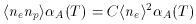(10)

(assuming T is constant in space), where the brackets are the space average of the product of the local proton and electron number densities, and the factor C > 1 takes into account the degree of clumpiness of the IGM. If ionized gas with electron density ne density filled uniformly a fraction 1/C of the available volume, the rest being empty space, the mean square density would be < ne2 > = ne2 / C = < ne >2 C.

The IGM is completely reionized when one ionizing photon has been emitted for each H atom by the radiation sources, and when the rate of emission of UV photons per unit (comoving) volume balances the radiative recombination rate, so that hydrogen atoms are photoionized faster than they can recombine. The complete reionization of the Universe manifests itself in the absence of an absorption trough in the spectra of galaxies and quasars at high redshifts. If the IGM along the line-of-sight to a distant source were neutral, the resonant scattering at the wavelength of the Ly(2p -> 1s; hP= 10.2 eV) transition of atomic hydrogen would remove all photons blueward of Lyoff the line-of-sight. For any reasonable density of the IGM, the scattering optical depth is so large that detectable absorption will be produced by relatively small column (or surface) densities of intergalactic neutral hydrogen.